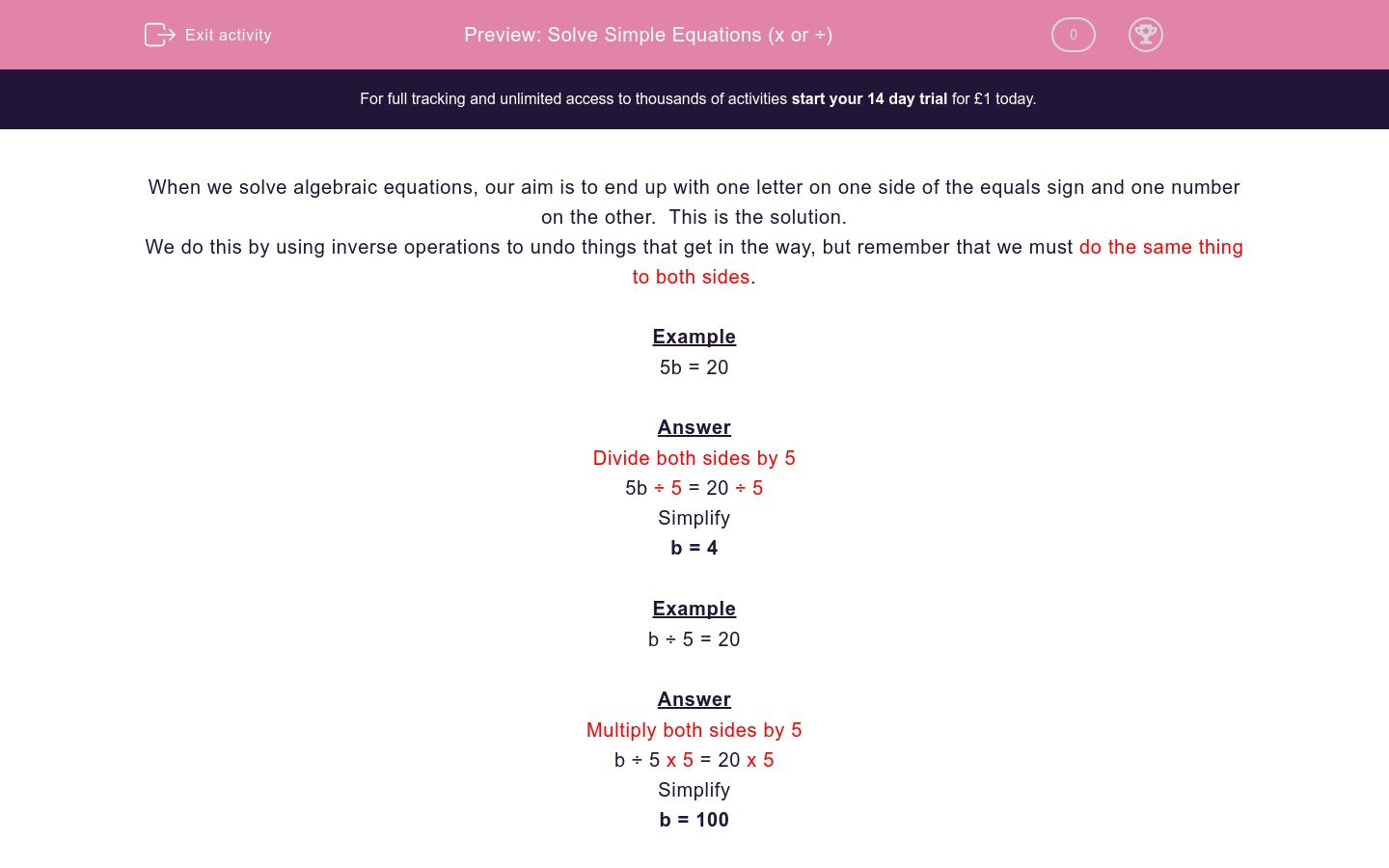# Solve Simple Equations (x or ÷)

In this worksheet, students solve simple one-stage equations that involve multiplication and divisionKey stage:  KS 2

Curriculum topic:   Maths and Numerical Reasoning

Curriculum subtopic:   Equations and Algebra

Difficulty level:### QUESTION 1 of 10

When we solve algebraic equations, our aim is to end up with one letter on one side of the equals sign and one number on the other.  This is the solution.

We do this by using inverse operations to undo things that get in the way, but remember that we must do the same thing to both sides.

Example

5b = 20

Divide both sides by 5

5b ÷ 5 = 20 ÷ 5

Simplify

b = 4

Example

b ÷ 5 = 20

Multiply both sides by 5

b ÷ 5 x 5 = 20 x 5

Simplify

b = 100

Solve for a:

5a = 15

a = 10

3

a = 3

Solve for a:

5a = 50

a = 10

45

a = 45

Solve for a:

a ÷ 5 = 50

a = 10

a = 250

a = 55

Solve for a:

a ÷ 3 = 12

a = 36

a = 4

a = 9

Solve for a:

a ÷ 4 = 12

a = 3

a = 48

a = 8

Solve for a:

a x 4 = 12

a = 3

a = 48

a = 8

Solve for a:

6a = 12

a = 6

a = 72

a = 2

Solve for a:

6a = 72

a = 36

a = 72

a = 12

Solve for a:

a ÷ 12 = 12

a = 144

a = 1

a = 0

Solve for a:

a ÷ 12 = 0

a = 144

a = 1

a = 0

• Question 1

Solve for a:

5a = 15

a = 3
EDDIE SAYS
5a means \'a times 5\' so we need to divide both sides by 5.

5 x a ÷ 5 = 15 ÷ 5

a = 3

• Question 2

Solve for a:

5a = 50

a = 10
EDDIE SAYS

5a means 'a times 5' so we need to divide both sides by 5.

5 x a ÷ 5 = 50 ÷ 5

a = 10

• Question 3

Solve for a:

a ÷ 5 = 50

a = 250
EDDIE SAYS

a ÷ 5 means 'a divided by 5' so we need to multiply both sides by 5.

a ÷ 5 x 5 = 50 x 5

a = 250

• Question 4

Solve for a:

a ÷ 3 = 12

a = 36
EDDIE SAYS

a ÷ 3 means 'a divided by 3' so we need to multiply both sides by 3.

a ÷ 3 x 3 = 12 x 3

a =36

• Question 5

Solve for a:

a ÷ 4 = 12

a = 48
EDDIE SAYS

a ÷ 4 means 'a divided by 4' so we need to multiply both sides by 4.

a ÷ 4 x 4 = 12 x 4

a =48

• Question 6

Solve for a:

a x 4 = 12

a = 3
EDDIE SAYS

4a means 'a times 4' so we need to divide both sides by 4.

4 x a ÷ 4 = 12 ÷ 4

a = 3

• Question 7

Solve for a:

6a = 12

a = 2
EDDIE SAYS

6a means 'a times 6' so we need to divide both sides by 6.

6 x a ÷ 6 = 12 ÷ 6

a = 2

• Question 8

Solve for a:

6a = 72

a = 12
EDDIE SAYS

6a means 'a times 6' so we need to divide both sides by 6.

6 x a ÷ 6 = 72 ÷ 6

a = 12

• Question 9

Solve for a:

a ÷ 12 = 12

a = 144
EDDIE SAYS

a ÷ 12 means 'a divided by 12' so we need to multiply both sides by 12.

a ÷ 12 x 12 = 12 x 12

a = 144

• Question 10

Solve for a:

a ÷ 12 = 0

a = 0
EDDIE SAYS

a ÷ 12 means 'a divided by 12' so we need to multiply both sides by 12.

a ÷ 12 x 12 = 0 x 12

a = 0

{Remember that if you have 12 lots of zero, you still have zero}
---- OR ----

Sign up for a £1 trial so you can track and measure your child's progress on this activity.

### What is EdPlace?

We're your National Curriculum aligned online education content provider helping each child succeed in English, maths and science from year 1 to GCSE. With an EdPlace account you’ll be able to track and measure progress, helping each child achieve their best. We build confidence and attainment by personalising each child’s learning at a level that suits them.

Get started